Tamilnadu State Board New Syllabus Samacheer Kalvi 9th Science Guide Pdf Chapter 11 Atomic Structure Text Book Back Questions and Answers, Notes.

## Tamilnadu Samacheer Kalvi 9th Science Solutions Chapter 11 Atomic Structure

### 9th Science Guide Atomic Structure Text Book Back Questions and AnswersI. Choose the correct answer :

Question 1.
Among the following the odd pair is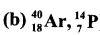Question 2.
Change in the number of neutrons in an atom changes it to
(a) an ion.
(b) an isotope.
(c) an isobar.
(d) another element.
(b) an isotopeQuestion 3.
The term nucleons refer to
(a) protons and electrons
(b) only neutrons
(c) electrons and neutrons
(d) protons and neutrons
(d) protons and neutrons

Question 4.
The number of protons, neutrons and electrons present respectively in $${ }_{35}^{80} \mathrm{Br}$$ are
(a) 80,80,35
(b) 35,55,80
(c) 35,35,80
(d) 35,45,35
(d) 35,45,35Question 5.
The correct electronic configuration of potassium is
(a) 2,8,9
(b) 2,8,1
(c) 2, 8, 8, 1
(d) 2, 8, 8, 3
(c) 2, 8, 8,1

II. State whether true or false. If false, correct the statement:

1. In an atom, electrons revolve around the nucleus in fixed orbits.
True.2. Isotopes of an element have different atomic numbers.
False.
Correct statement: Same atomic numbers but different mass numbers.

3. Electrons have negligible mass and charge.
True.

4. Smaller the size of the orbit, lower is the energy of the orbit.
True.

5. The maximum number of electron in L Shell is 10.
False.
Correct statement :The maximum number of electrons in L Shell is 8.III. Fill in the Blanks :

1. Calcium and Argon are examples of a pair of ………….
isobars

2. Total number of electrons that can be accommodated in an orbit is given by …………..
the formula 2n2

3. ………….isotope is used in the nuclear reactors.
Uranium-235

4. The number of neutrons present in $${ }_{3}^{7} \mathrm{Li}$$ is ………….
4

5. The valency of Argon is ………………
ZeroIV. Match the following :

 a) Dalton 1. Hydrogen atom model b) Chadwick 2. Discovery of nucleus c) Rutherford 3. First atomic theory d) Neils Bohr 4. Plum pudding model 5. Discovery of neutrons

a – 3, b – 5, c – 2, d – 1.

V. Complete the following table :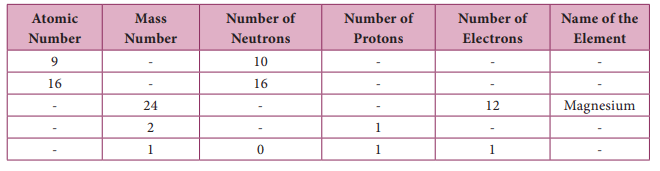Question 1.
Name an element which has the same number of electrons in its first and second shell.
Beryllium. Atomic number – 4 (K-shell-2, L-shell-2)Question 2.
Write the electronic configuration of K and ClQuestion 3.
Write down the names of the particles represented by the following symbols and explain the meaning of superscript and subscript numbers attached. 1H1, 0n1, -1C0
1H1 – hydrogen atom mass number 1. Atom number 1.
0n1– neutron change 0. Mass 1 amu.
-1C0 – electron change -1. Mass negligible.

Question 4.
For an atom ‘X’, K, L, and M shells are completely filled. How many electrons will be present in it?
K – 2, L – 8, M – 18, 28 electrons – NickelQuestion 5.
What is the same about the electron structures of:
a. Lithium, Sodium and Potassium.
b. Beryllium, Magnesium and Calcium.
a. Lithium, sodium & potassium have 1 electron in their outermost shell.
b. Beryllium, magnesium and calcium have 2 electrons in their outermost shell.

Question 1.
How was it shown that atom has empty space?
Rutherford performed an experiment of bombarding a thin gold foil with very small positively charged particles called a particles. He observed that most of the alpha particles passed straight through the foil. He infered by thin experiment that most of the space in the atom is empty.

Question 2.
Why do $${ }_{17}^{35} \mathrm{Cl}$$ and $${ }_{17}^{37} \mathrm{Cl}$$ have the same chemical properties? In what respect do these atoms differ?
$${ }_{17}^{35} \mathrm{Cl}$$ and $${ }_{17}^{37} \mathrm{Cl}$$ have same chemical properties because they have same number of electrons but their atoms differ in the number of neutrons.Question 3.
Draw the structure of oxygen and sulphur atoms.Question 4.
Calculate the number of neutrons, protons and electrons :
(i) atomic number 3 and mass number 7
(ii) atomic number 92 and mass number 238.
(i) atomic number 3 and mass number 7
∴ No. of electrons (or) No. of protons = 3
Mass number = No. of protons + No. of neutrons
∴ No. of neutrons = 7-3=4

(ii) atomic number 92 and mass number 238
Atomic number = 92
∴ No. of electrons = 92
No. of protons = 92
No. of neutrons = 238-92 = 146Question 5.
What are nucleons? How many nucleons are present in Phosphorous? Draw its structure.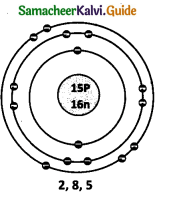The number of protons and neutrons present in the nucleus are called nucleons. The number of nucleons present is phosphorus is 31.

Question 1.
What conclusions were made from the observations of Gold foil experiment?

In Rutherford’s Alpha ray scattering experiment, the following conclusions were made from the observations.

• Atom has a very small nucleus at the centre.
• There is a large empty space around the nucleus.
• The entire mass of an atom is concentrated in a very small positively charged region, which is called Nucleus.
• The electrons are distributed in the vacant space around the nucleus.
• The electrons move in circular paths around the nucleus.

Question 2.
Explain the postulates of Bohr’s atomic model.
The main postulates are:

• In atoms, electrons revolve around the nucleus in stationary circular paths called orbits or shells or energy levels.
• While revolving in orbits the electrons do not radiate energy.
• The circular orbits are numbered as 1,2,3,4,… or designated as K, L, M, N, shells. These numbers are referred to as principal quantum numbers (n).
• K shell (n=l) is closest to the nucleus and is associated with lowest energy.
• The energy of each orbit or shell is a fixed quantity and the energy is quantized.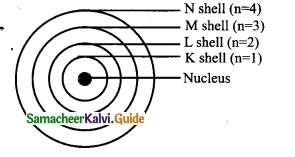Question 3.
State Gay Lussac’s law of combining volumes, explain with an illustration.
Whenever gases react together, the volumes of the reacting gases as well as the products, if they are gases, bear a simple whole number ratio, provided all the volumes are measured under similar conditions of temperature and pressure.
Example:
Step 1: Hydrogen combines with oxygen to form water (word equation) Hydrogen + Oxygen → Water.i. e. Two volumes of hydrogen reacts with one volume of oxygen to form two volumes of water vapour, i.e. the ratio by volume which gases bears is 2:1:2 which is a simple whole number ratio.
It follows that at a given temperature and pressure, the volumes of all gaseous reactants and products bear a simple whole number ratio to each other.

Intext Activities

ACTIVITY – 1

Symbolically represent the following atoms using the atomic number and mass number, a) Carbon b) Oxygen c) Silicon d) BerylliumACTIVITY – 2

Assign the valency for Phosphorus, Chlorine, Silicon and Argon
Phosphorus – P – 3, 5
Chlorine – Cl – 1
Silicon – Si – 4
Argon – Ar – 0

ACTIVITY – 4

Draw the structures of the isotopes of oxygen O16 and O18
Atomic number of oxygen = 8.ACTIVITY – 5

Draw the model of the following pairs of isotones:
(i) Fluorine & Neon (ii) Sodium & Magnesium (iii) Aluminum and Silicon.ACTIVITY – 6

Nitrogen combines with hydrogen to form ammonia (NH3). Illustrate Gay Lussac’s law using this example.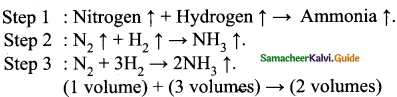1 volume of nitrogen reacts with 3 volumes of hydrogen to form 2 volumes of ammonia gas. The ratio by volume which gases bear is 1 : 3 : 2. Which is a simple while number ratio.Test Yourself :

Question 1.
Calculate the number of neutrons in the following atoms: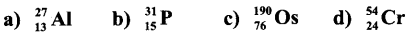Number of neutrons (n) = Mass number (A) – Atomic number (z)
a) $$\frac{27}{13} \mathrm{Al}$$ ⇒ Number of neutrons (n) = 27 – 13 = 14
b) $$\frac{31}{15} \mathrm{P}$$ ⇒ Number of neutrons (n) = 31-15 = 16
c) $$\frac{190}{76} \mathrm{Os}$$ ⇒ Number of neutrons (n) = 190 – 76 = 114
d) $$\frac{54}{24} \mathrm{Cr}$$ ⇒ Number of neutrons (n) = 54 – 24 = 30

I. Choose the correct answer :

Question 1.
Hydrogen atom does not have …………………..
(a) Proton
(b) Neutron
(c) Electron
(d) Proton and electron
(b) Neutron

Question 2.
An electron has ……………………
(a) Mass 1/1837th of proton and positively charged
(b) Mass equal to proton and positively charged.
(c) Mass 1 / 1837th of proton and negatively charged.
(d) Mass equal to proton and negatively charged.
(c) Mass 1/1837thof proton and negatively chargedQuestion 3.
Which statement is not correct about isotopes of an element?
(a) Their chemical properties are same.
(b) Their atomic number is same.
(c) Number of electrons are different.
(d) Number of neutrons are different.
(c) Number of electrons are different

Question 4.
Co-60 is used in the treatment of …………………..
(a) Cancer
(b) Thyroid disorders
(c) Leukemia
(d) Blockage of arteries.
(a) CancerQuestion 5.
Every atom has an equal number of protons and electrons. The nature of the atom is …………………..
(a) Positive
(b) Neutral
(c) Negative
(d) None of the above
(b) Neutral

Question 6.
Gold foil alpha particle scattering experiment was performed by …………………..
(a) Dalton
(b) Goldstein
(c) Thomson
(d) Rutherford
(d) Rutherford

Question 7.
Which subatomic particles have no charge …………………..
(a) Proton
(b) Neutron
(c) Electron
(d) Proton and electron
(b) NeutronQuestion 8.
The outermost shell of an atom cannot accommodate electrons more than
(a) 2
(b) 8
(c) 18
(d) 32
(b)8

Question 9.
The ratio of masses of oxygen in CO and C02 for fixed mass of carbon is 1 : 2. The above is the law of ……………………
(a) Law of multiple proportions
(b) Law of reciprocal proportions
(c) Gay Lussac’s Law of combining volumes
(d) None of the above
Ans:
(a) Law of multiple proportions

Question 10.
………………….. is a radioactive isotope present in our bodv.
(a) Cobalt 60
(b) Uranium 235
(c) Iodine 131
(d) Potassium 40
(d) Pottassium 40

Question 11.
Proton was discovered by ………………….
(a) Rutherford
(b) Goldstein
(d) J.J. Thomson
(a) RutherfordQuestion 12.
Electron was discovered by …………………..
(a) J.J. Thomson
(b) Rutherford
(c) Goldstein
(a) J.J. Thomson

Question 13.
α – particles are …………………..
(a) Negative charged particles
(b) Positive charged particles
(c) Beam of Neutrons
(a) Negative charged particles

Question 14.
An α – particle is …………………..
(a) hydrogen Nucleus
(b) a Helium Nucleus
(c) a Proton
(d) an electron
(b) a He Nucleus

Question 15.
The electronic configuration of an atom is 2,8,3. The valency of the atom is ……………………
(a) 13
(b) 10
(c) 3
(d) 8
(c) 3Question 16.
The No. of electrons in the outermost shell of the atom of an inert gas ……………………
(a) 0
(b) 1
(c) 2
(d) 8
(d) 8

Question 17.
Which one of the following will have the maximum charge / mass ratio?
(a) Electron
(b) Proton
(c) Neutron
(d) a – Particle
(a) Electron

Question 18.
The maximum No. of electrons that can be accommodated in M shell is ……………………
(a) 2
(b) 8
(c) 18
(d) 32
(c) 18

Question 19.
Elements with valency 1 are ……………………
(a) always metals
(b) always metaloids
(c) either metals (or) non-metals
(d) always non – metals
(a) always metals

Question 20.
The electronic configuration of ‘Cl’ is ……………………
(a) 2,7
(b) 2,8,8,7
(c) 2,8,7
(d) 2,7,8
(c) 2, 8, 7Question 21.
The isotope used to remove the brain tumour and treatment of Cancer is ……………………
(a) Cobalt 60
(b) Uranium 235
(c) Iodine 131
(d) Na – 24
(a) Cobalt 60

II. Fill in the Blanks :

1. ………………….. are the building blocks of matter.
Atoms

2. The combination of different elements to form a compound is governed by certain basic rules called …………………..
Laws of chemical combination

3. Volume of the nucleus of an atom is ………………….. compared to the volume of the extranuclear part of the atom.
very small

4. the Atomic number of an element is 20. It has………………….. valence electrons.
2

5. Atoms are so tiny their mass number cannot be expressed in grams but expressed in…………………..
atomic mass unit (amu)

6. Elements having completely filled outermost shell, show………………….. valency.
Zero

7. A ………………….. number describes a specific aspect of an electron.
quantum8. When an atom gives electrons from its outermost shell, it becomes ………………….. charged.
positively

9. The elementary particles such as protons and neutrons are collectively called as…………………..
nucleons

10. In two isotopic species number of protons is same but the number of………………….. is different.
Neutrons

11. The combination of different elements form a…………………..
Compound

12. A compound is governed by basic rules are known as ……………………
Laws of chemical combination

13. The ratio of masses of H and O in H2O is…………….
1 : 8

14. α, β, γ rays are during the ratio active decay of an atom.
emitted15. Beryllium + α – rays → Carbon +……………….
Neutron

16. …………… = No. of protons = No. of the electrons.
Atomic No.

17. ‘A’ is denoted by …………………
Mass No

18. ‘Z’ is denoted by . …………………
Atomic No

19. Mass No =………………… + No. of Neutrons
No. of protons20. The Mass No. of 27 is for the element…………………….
Aluminium

21. U-235 Isotope is used as ………………….
Fuel in nuclear reactors

22. The symbol of phosphorus is ……………………….
P

23. Na – →……………….. Na+.
e-

24. Elements with 4 to 7 in their valence shells are …………………
non -metals25. Valency of the elements having ……………..is 1, 2, 3 respectively.
Valence electrons 1, 2, 3

26. Atomic orbitals allow atoms to make…………………….. bonds
Co-valent

27. The symbol of Azimuthal quantum number is……………………..
l

28. The symbol of the Principal quantum number is……………………..
n

29. Valency is the combining capacity of ……………………..
an atomIII. State whether true or false. If false, correct the statement:

1. In Rutherford’s experiment, bouncing of alpha particles suggests that nucleus of an atom is negatively charged.
False
Correct statement: Nucleus of an atom is positively charged.

2. Electrons revolve around the nucleus in definite open shells.
False
Correct statement: Electrons revolve around the nucleus in definite closed shells.

3. K and L shell can accommodate a maximum 2 and 8 electrons respectively.
True

4. Radio activity of Carbon 14 is considered to determine the age of object, especially of archeological importance.
True

5. In a neutral atom number of protons is equal to the number of neutrons.
Correct statement: In a neutral atom number of protons is equal to the number of
electrons.

6. If an atom has 6 electrons in its outermost shell, it is inert.
Correct statement: If an atom has 8 electrons in its outermost shell, it is inert.7. The valency of Chlorine is 7.
Correct statement: The valency is 1.

8. Smaller the size of the orbit, the smaller the energy of the orbit.
True

9. The stability of the nucleus is determined by the Neutron – Proton ratio.
True10. Isotopes of elements have the same chemical properties.
True

IV. Match the following :

1.

 Column A Column B 1) Prinicipal quantum number a) Orientation of orbitals 2) Azimuthal quantum number b) Spin of the electron 3) Magnetic quantum number c) Main energy level 4) Spin quantum number of orbital d) Sub shell/shape of orbital

1) – c, 2) – d, 3) – a, 4) – b2.

 Column A Column B 1. K shell a) 8 2. L shell b) 32 3. M shell c) 2 4. N shell d) 18

1) – c, 2) – a, 3) – d, 4) – b

3.

 Column A Column B a) Mass of proton 1. +1 b) Mass of electron 2. -1 c) Charge of electron 3. 9.31 x 10-28g d) Charge of proton 4. 1.67 x 10-24g

a) 4
b) 3
c) 2
d) 1

Question 1.
If and element M has mass number 24 and atomic number 12, how many neutrons does its atom contain?
Mass number = No. of protons + neutrons
Atomic number = No. of protons
No. of neutrons = 24 – 12 = 12.

Question 2.
Atomic number of A and B are 18 and 19 respectively. What is the electronic configuration of A and B?
Electronic configuration of A = 2, 8, 8
Electronic configuration of B = 2, 8, 8, 1.

Question 3.
How will you define the term ‘electronic configuration’?
The systematic arrangement of electrons in various shells or orbits in an atom is called the electronic configuration.

Question 4.
Why do the chemical properties of an element are determined by valence electrons?
Because they are the one which takes part in the chemical reaction.

Question 5.
What are the elements which have valence electrons 1 or 2 or 3 are called and what are the elements with valence Electrons 4 to 7 in their valence shells?
Elements with valence electrons 1 or 2 or 3 are metals and valence electrons with 4 to 7 are non – metals.

Question 6.
How is an atom of the element represented? Give an example.
If ‘X’ is the element, atomic mass at the upper left side and atomic number at the lower left side of the symbol of the element.
Example : $${ }_{11}^{23} \mathrm{Na}$$ ⇒ 23 →Atomic Mass, 11 →Atomic number.

Question 7.
Why an atom is considered neutral?
An atom of an element contains equal number of positively charged protons and negatively charged electrons and so it is neutral.

Question 8.
Name the scientist and his experiment to prove that nucleus of an atom is positively charged.
Name of the scientist – Earnest Rutherford
Name of the experiment – Gold foil alpha particle scattering experiment.

Question 9.
Name the particles which determine the mass of an atom?
The mass of an atom is determined by protons and neutrons.
Atomic mass = No. of protons + No. of neutrons.

Question 10.
What are the shells?
Different energy levels outside the nucleus are called as shells. They are designated as K, L, M, N and numbered as 1, 2, 3…..

Question 11.
Rutherford’s model of an atom has a very small ………….. at the center.
Nucleus.

Question 12.
Niels Bohr was Born on …………
October 7, 1885.

Question 13.
When an electron returns from high energy level to lower energy level it gives …………
It gives off energy.

Question 14.
In $${ }_{\mathrm{Z}}^{\mathrm{A}} \mathrm{X}$$what are the letters A, Z, X stand for?
X → symbol of element
Z →Atomic No.
A → Mass No.

Question 1.
State the law of reciprocal proportion.
If two different elements combine separately with the same weight of a third element, the ratios of the masses in which they do so are either the same or a simple multiple of the mass ratio in which they combine.

Question 2.
Why proton is considered to be the fingerprint of an atom?
An atom of an element has its own characteristic number of protons in its nucleus, which distinguishes it from the atoms of other elements. Hence proton is considered to be the fingerprint of an atom.
This characteristic number (Number of protons) is called the atomic number of the element. The atomic number is denoted by Z.

Question 3.
How can an element be identified on the Periodic Table? What makes an element different from another element?
An element can be identified by its atomic number which is a representation of the number of protons contained in the nucleus of a particular element. The amount of protons of an element never changes.

Question 4.
Because atoms are so small, what do scientists do?
Since atoms are so small, scientists create models to describe them. A model may be a diagram, mental picture, a mathematical statement or an object that helps explain ideas about the natural world.

Question 5.
What are isotopes?
Atoms of the same element with the same number of protons and a different number of neutrons – the number of protons will never change! Ex.: Carbon 12, Carbon 13 and Carbon 14 are isotopes of the element carbon.

Question 6.
Draw the diagram of laws of chemical combination.
Laws of chemical combinationQuestion 7.
Define Isobars.
Isobars are the atoms of the different elements of same mass number but different atomic number.

Question 8.
What is a combination reaction?
A combination reaction is a reaction where two or more substances combine to form a single substance.
The combination of different elements to form a compound is governed by certain basic rules. These rules are known as Laws of chemical combination.

Question 9.
What are the salient features of Rutherford’s model of an atom?
RUTHERFORD’S MODEL OF AN ATOM- Salient features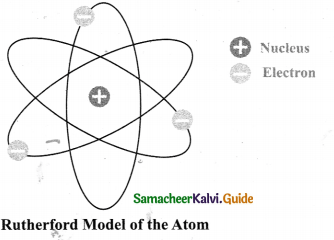• Atom has a very small nucleus at the center.
• There is large empty space around the nucleus.
• Entire mass of an atom is concentrated in a very small positively charged region which is called the nucleus.
• Electrons are distributed in the vacant space around the nucleus.
• The electrons move in circular paths around the nucleus.

Question 10.
What are the Limitations of Bohr’s Model?
Limitations of Bohr’s model:

• One main limitation was that his model was applicable only to Hydrogen.
• It could not be extended to multi-electron atoms.
• Hence more research and deeper study of atoms became necessary.

Question 11.
Explain the Composition of the Nucleus.
COMPOSITION OF NUCLEUS

• Electrons have a negligible mass; hence the mass of the atom mainly depends on the mass of the nucleus. Nucleus of an atom consists of two components, they are protons and neutrons.
• Protons are positively charged. Protons repel each other because of their like charges. Hence more than one proton cannot be packed in a small volume to form a stable nucleus, unless neutrons are present.
• Neutrons reduce the repulsive force between the positively charged protons and contribute to the force that holds the particles in the nucleus together.

Question 12.
Define the Nucleons.
Nucleons: The elementary particles such as protons and neutrons are collectively called as Nucleons.

Question 13.
What are the relation between mass no. & atomic no?
Relationship between Mass Number and Atomic Number:
Mass Number (A) = Number of Protons + Number of Neutrons(n)
Atomic Number (Z) = Number of Protons or Number of Electrons
Mass Number = Atomic Number (Z) + Number of neutrons (n)
A = Z + n.

Question 14.
What are the types of Quantum Number?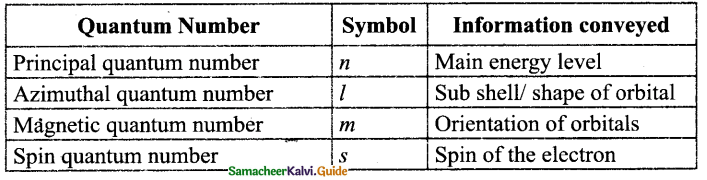Question 15.
Draw the atomic structure of Mg (At. No. 12, Mass No. 24)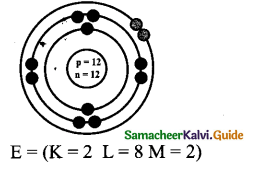Question 16.
Compare the charge and mass of protons and electrons.Question 17.
The atomic number of Calcium is 20. It has K = 2, L = 8, M = 8, N = 2.
If we remove the last 2 electrons, that is from N shell, Ca2+ and we will be having 8 in the outermost shell (M shell). So we have completely filled outer shell. (The maximum 8 electrons in a shell is a completely filled shell).

Question 18.
State the law of multiple proportion. .
When two elements A and B combine together to form more than one compound, then masses of A which separately combines with a fixed mass of B are in simple ratio.

Question 19.
List the uses of isotopes.
Uranium 235 – Used as fuel in nuclear reactors
Cobalt 60 – Used in the treatment of cancer
Iodine 131 – Used in the treatment of goitre.
Carbon 14 – To find the age of plants and animals.

Question 20.
What is isotone? Give an example.
Atoms of different elements with different atomic numbers and different mass numbers, but with the same number of neutrons are called isotones.
Example: Pair of elements Boron and Carbon has the same number of neutrons but
different number of protons and hence different atomic numbers.

Question 1.
Prove that in the following example, Sulphur and Oxygen combine using the law of reciprocal proportions.
Oxygen and sulfur react with copper to give copper oxide and copper sulfide, respectively. Sulphur and oxygen also react with each other to form S02. Therefore,

in CuS ⇒ Cu : S = 63.5 : 32
in CuO ⇒ Cu : O = 63.5 : 16
S : 0 = 32 : 16 ⇒ S:0= 2:1
Now in SO2:
S : 0 = 32 : 32 ⇒ S: 0 = 1:1
Thus the ratio between the two ratios is 2 : 1
This is a simple multiple ratio.

Question 2.
When the nucleus of an atom possesses either too many or too few neutrons compared to the number of protons it becomes unstable. These are called radioactive isotopes. Unstable nuclei split up in a process called radioactive decay and emit radioactive radiation.

Many elements have isotopes of which some of them are radioactive isotopes. There is a lot of low-level natural radioactivity around us. For example, our bodies contain radioisotopes, such as Potassium – 40, which continuously emit radiation, but because the amount is very low this does not harm us. Carbon -14 is a radioactive isotope used to date organic material.

Question 3.
Describe the limitations in Rutherford’s model.

• According to electromagnetic theory, a moving electron, accelerate and continuously lose energy.
• Due to the loss of energy, path of the electron may reduce and finally the electron fall into the nucleus.
• If it happens so, atom becomes unstable. But atom are stable. Thus Rutherford’s model failed to explain the stability of an atom.

Question 4.
Explain the electronic configuration of atoms with an illustration.
Ans. Electronic configuration of atoms:

• Electrons occupy different energy levels called orbits or shells.
• The distribution of electrons in these orbits of an atom is governed by certain rules or conditions. These are known as Bohr and Bury Rules of electronic configuration.
• Bohr and Bury simultaneously proposed the following rules for the distribution of electrons in different shells.

Rule 1: The maximum number of electrons that can be accommodated in a shell is equal to 2n2 where ‘n’ is the quantum number of the shell (i.e., the serial number of the shell from the nucleus).
Shell Value of (n) Maximum number of electrons (2n2)

 Shell Value of (n) Maximum number of electrons (2n2) K 1 2 x 12 = 2 L 2 2 x 22 = 8 M 3 2 x 32 = 18 N 4 2 x 42 = 32

Rule 2: Shells are filled in a stepwise manner in the increasing order of energy.
Rule 3: The outermost shell of an atom cannot have more than 8 electrons even if it has the capacity to accommodate more electrons. For example Electronic configuration in Ca

Illustration: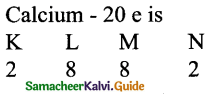Question 5.
Explain the valence electron and valency with an illustration.Valence elect runs
How many electrons are in the outermost shell of nitrogen? 5 The outermost shell of an atorh is called its valence shell and the electrons present in the valence shell are known as valence electrons.

The chemical properties of elements are decided by these valance electrons, since they are the ones that take part in chemical reaction.

The elements with same number of electrons in the valence shell show similar properties and those with a different number of valence electrons show different chemical properties. Elements, which have valence electrons 1 ‘ or 2 or 3 (except Hydrogen) are metals.

These elements can lose electrons to form ions which are positive charged and are called cations.Elements with 4 to 7 electrons in their valence shells are non-metals.
These elements can gain electrons to form ions which are negatively charged and are called anionsVALENCY:

Valency of the element is the combining capacity of the element with other elements and is equal to the number of electrons that take part in a chemical reaction. The valency of the elements having valence electrons 1, 2, 3 is 1, 2, 3 respectively.
While valency of element with 4, 5, 6 & 7 valence electron is 4, 3, 2 and 1 (8 – valence electrons) respectively. Elements having completely filled the outermost shell show Zero valency.

For example The electronic configuration of Neon is 2,8 (completely filled). So valency is 0

Illustration:
Assign the valency of Magnesium & Sulphur.
Electronic configuration of magnesium is 2, 8, 2. So valency is 2.
The electronic configuration of sulphur is 2, 8, 6. So valency is 2 i.e. (8 – 6).